# Divide by N clock

13 Dec 2011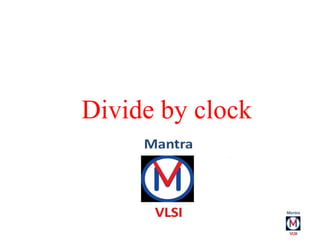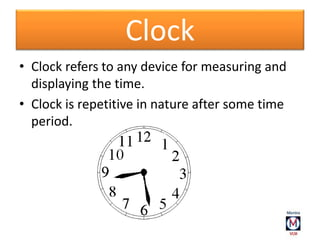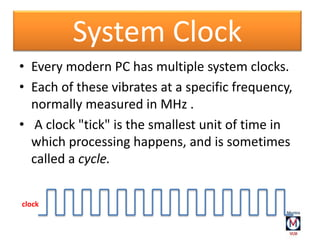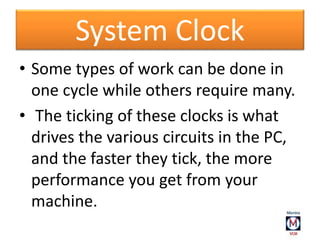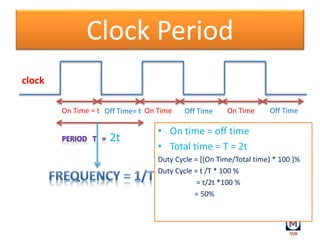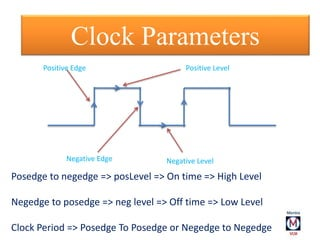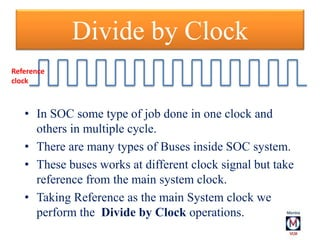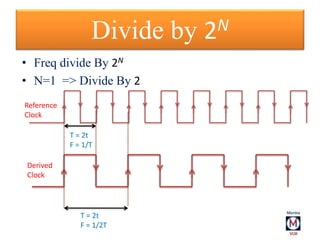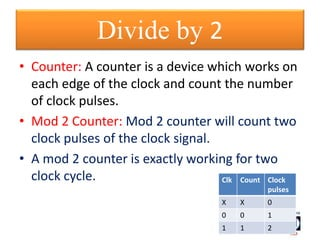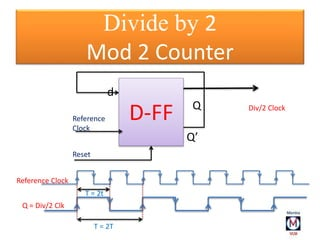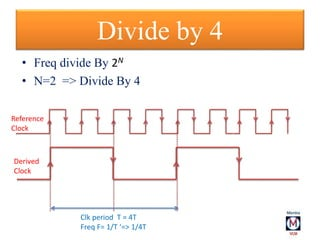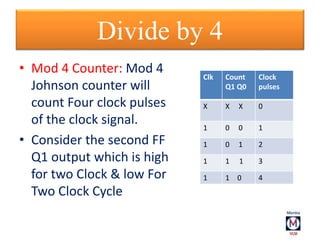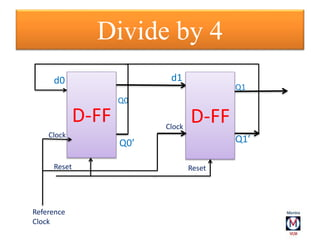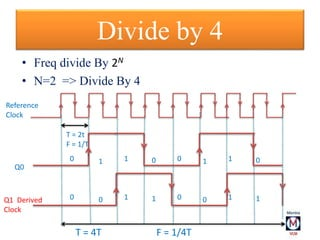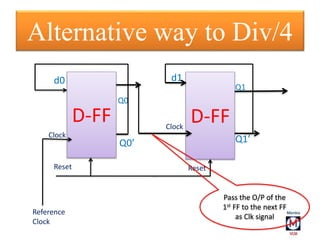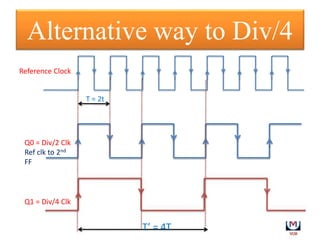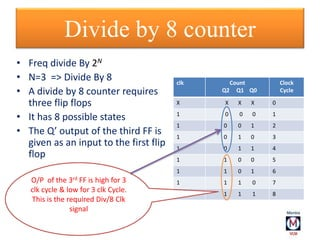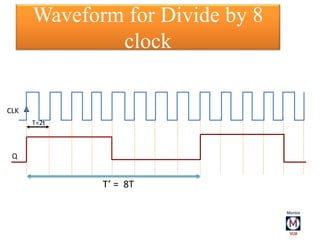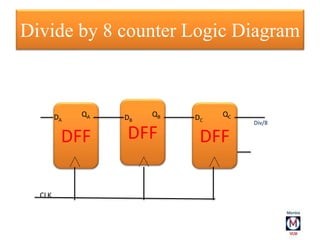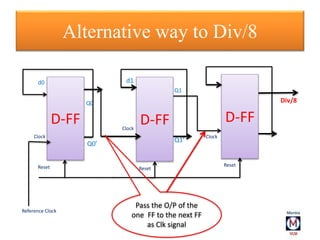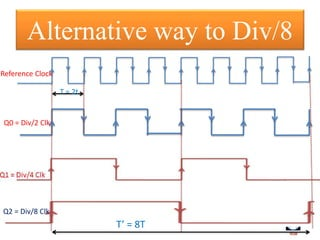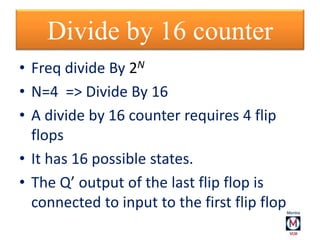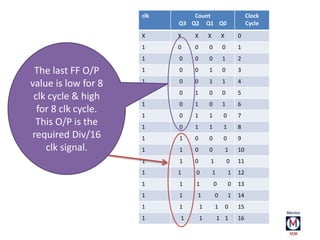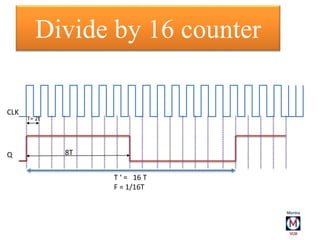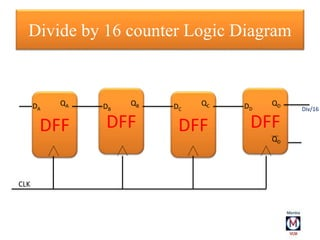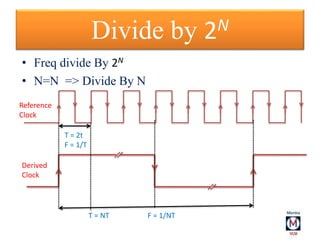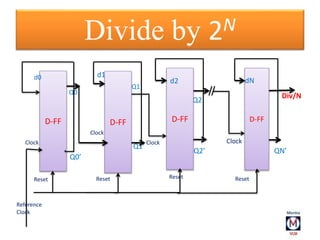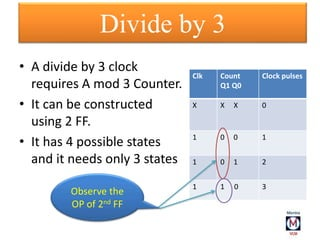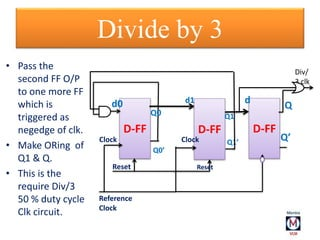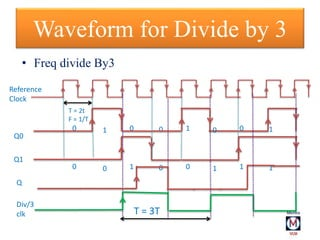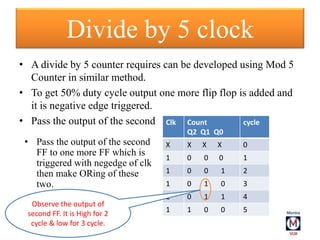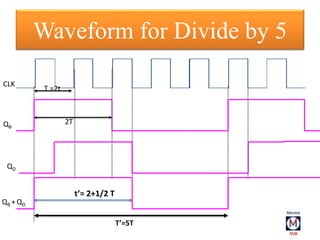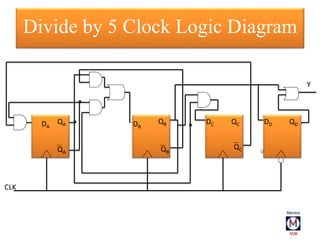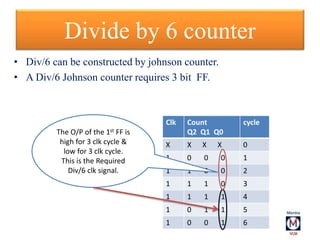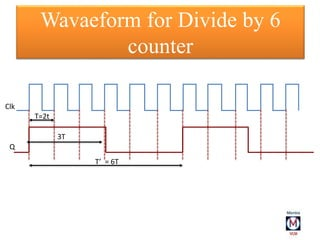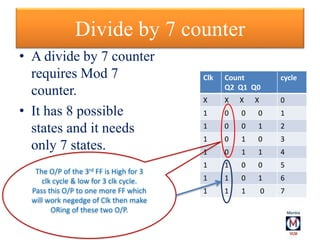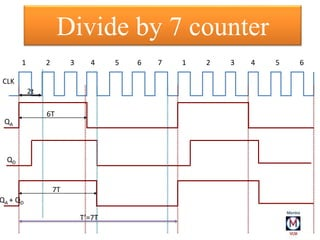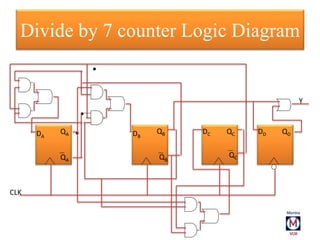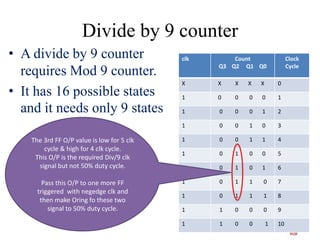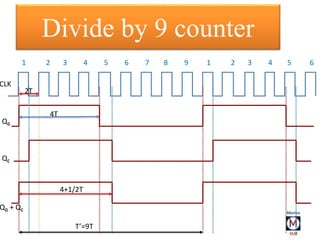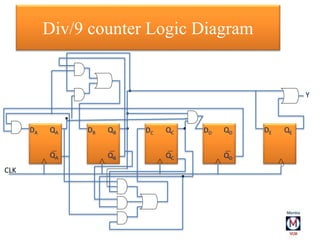1 sur 42

### Divide by N clock

• 1. Divide by clock Deepak Floria deepakfloria@gmail.com
• 2. Clock • Clock refers to any device for measuring and displaying the time. • Clock is repetitive in nature after some time period.
• 3. • Every modern PC has multiple system clocks. • Each of these vibrates at a specific frequency, normally measured in MHz . • A clock "tick" is the smallest unit of time in which processing happens, and is sometimes called a cycle. System Clock clock
• 4. • Some types of work can be done in one cycle while others require many. • The ticking of these clocks is what drives the various circuits in the PC, and the faster they tick, the more performance you get from your machine. System Clock
• 5. Clock Period clock Off Time= tOn Time = t On Time On TimeOff Time Off Time 2t • On time = off time • Total time = T = 2t Duty Cycle = [(On Time/Total time) * 100 ]% Duty Cycle = t /T * 100 % = t/2t *100 % = 50%
• 6. ` Positive Level Negative Level Positive Edge Negative Edge Clock Parameters Posedge to negedge => posLevel => On time => High Level Negedge to posedge => neg level => Off time => Low Level Clock Period => Posedge To Posedge or Negedge to Negedge
• 7. Divide by Clock • In SOC some type of job done in one clock and others in multiple cycle. • There are many types of Buses inside SOC system. • These buses works at different clock signal but take reference from the main system clock. • Taking Reference as the main System clock we perform the Divide by Clock operations. Reference clock
• 8. Divide by 2N • Freq divide By 2N • N=1 => Divide By 2 T = 2t F = 1/T T = 2t F = 1/2T Reference Clock Derived Clock
• 9. • Counter: A counter is a device which works on each edge of the clock and count the number of clock pulses. • Mod 2 Counter: Mod 2 counter will count two clock pulses of the clock signal. • A mod 2 counter is exactly working for two clock cycle. Divide by 2 Clk Count Clock pulses X X 0 0 0 1 1 1 2
• 10. Q D-FF d Q’ Reference Clock Reset Divide by 2 Mod 2 Counter T = 2t T = 2T Reference Clock Q = Div/2 Clk Div/2 Clock
• 11. Divide by 4 • Freq divide By 2N • N=2 => Divide By 4 Reference Clock Derived Clock Clk period T = 4T Freq F= 1/T ‘=> 1/4T
• 12. • Mod 4 Counter: Mod 4 Johnson counter will count Four clock pulses of the clock signal. • Consider the second FF Q1 output which is high for two Clock & low For Two Clock Cycle Divide by 4 Clk Count Q1 Q0 Clock pulses X X X 0 1 0 0 1 1 0 1 2 1 1 1 3 1 1 0 4
• 13. Divide by 4 D-FF d0 Q0’ d1 D-FFClock Reset Reference Clock Reset Q0 Q1 Clock Q1’
• 14. Divide by 4 • Freq divide By 2N • N=2 => Divide By 4 Reference Clock Q1 Derived Clock Q0 T = 2t F = 1/T T = 4T F = 1/4T 0 0 1 0 1 1 0 1 0 0 1 0 1 1 0 1
• 15. D-FF d0 Q0’ d1 D-FFClock Reset Reference Clock Reset Q0 Q1 Clock Q1’ Alternative way to Div/4 Pass the O/P of the 1st FF to the next FF as Clk signal
• 16. Alternative way to Div/4 Q1 = Div/4 Clk T = 2t T’ = 4T Reference Clock Q0 = Div/2 Clk Ref clk to 2nd FF
• 17. Divide by 8 counter • Freq divide By 2N • N=3 => Divide By 8 • A divide by 8 counter requires three flip flops • It has 8 possible states • The Q’ output of the third FF is given as an input to the first flip flop clk Count Q2 Q1 Q0 Clock Cycle X X X X 0 1 0 0 0 1 1 0 0 1 2 1 0 1 0 3 1 0 1 1 4 1 1 0 0 5 1 1 0 1 6 1 1 1 0 7 1 1 1 1 8 O/P of the 3rd FF is high for 3 clk cycle & low for 3 clk Cycle. This is the required Div/8 Clk signal
• 18. Waveform for Divide by 8 clock T=2t T’ = 8T CLK Q
• 19. Divide by 8 counter Logic Diagram DFF DFFDFF CLK DA QA DB DC QB QC Div/8
• 20. Alternative way to Div/8 D-FF d0 Q0’ d1 D-FFClock Reset Reference Clock Reset Q0 Q1 Clock Q1’ D-FF Clock Reset Div/8 Pass the O/P of the one FF to the next FF as Clk signal
• 21. Alternative way to Div/8 Q2 = Div/8 Clk T = 2t T’ = 8T Reference Clock Q0 = Div/2 Clk
• 22. Divide by 16 counter • Freq divide By 2N • N=4 => Divide By 16 • A divide by 16 counter requires 4 flip flops • It has 16 possible states. • The Q’ output of the last flip flop is connected to input to the first flip flop
• 23. clk Count Q3 Q2 Q1 Q0 Clock Cycle X X X X X 0 1 0 0 0 0 1 1 0 0 0 1 2 1 0 0 1 0 3 1 0 0 1 1 4 1 0 1 0 0 5 1 0 1 0 1 6 1 0 1 1 0 7 1 0 1 1 1 8 1 1 0 0 0 9 1 1 0 0 1 10 1 1 0 1 0 11 1 1 0 1 1 12 1 1 1 0 0 13 1 1 1 0 1 14 1 1 1 1 0 15 1 1 1 1 1 16 The last FF O/P value is low for 8 clk cycle & high for 8 clk cycle. This O/P is the required Div/16 clk signal.
• 24. Divide by 16 counter CLK Q T= 2t 8T T ‘ = 16 T F = 1/16T
• 25. Divide by 16 counter Logic Diagram DFF DFF DFFDFF CLK DA QA DB DC DD QB QC QD QD Div/16
• 26. Divide by 2N • Freq divide By 2N • N=N => Divide By N T = 2t F = 1/T T = NT F = 1/NT Reference Clock Derived Clock
• 27. Divide by 2N Q2 D-FF d0 Q0’ d1 D-FF Clock Reset Reference Clock Reset Q0 Q1 Clock Q1’ D-FF Clock Reset Div/N D-FF Reset Clock Q2’ QN’ d2 dN
• 28. • A divide by 3 clock requires A mod 3 Counter. • It can be constructed using 2 FF. • It has 4 possible states and it needs only 3 states Divide by 3 Clk Count Q1 Q0 Clock pulses X X X 0 1 0 0 1 1 0 1 2 1 1 0 3 Observe the OP of 2nd FF
• 29. Div/ 3 clk D-FFD-FF d0 Q0’ d1 D-FF Clock Reset Reference Clock Reset Q0 Q1 Clock Q1’ d Q Q’ Divide by 3 • Pass the second FF O/P to one more FF which is triggered as negedge of clk. • Make ORing of Q1 & Q. • This is the require Div/3 50 % duty cycle Clk circuit.
• 30. Waveform for Divide by 3 • Freq divide By3 Reference Clock Q1 Q0 T = 2t F = 1/T T = 3T 0 0 1 0 0 1 0 0 1 0 0 1 0 1 1 1 Q Div/3 clk
• 31. • A divide by 5 counter requires can be developed using Mod 5 Counter in similar method. • To get 50% duty cycle output one more flip flop is added and it is negative edge triggered. • Pass the output of the second Divide by 5 clock Clk Count Q2 Q1 Q0 cycle X X X X 0 1 0 0 0 1 1 0 0 1 2 1 0 1 0 3 1 0 1 1 4 1 1 0 0 5 Observe the output of second FF. It is High for 2 cycle & low for 3 cycle. • Pass the output of the second FF to one more FF which is triggered with negedge of clk then make ORing of these two.
• 32. 2T Waveform for Divide by 5 T =2t t’= 2+1/2 T T’=5T CLK QB QD QB + QD
• 33. QA QA QB QB QC QC QDDA DB DC DD . . CLK Y . Divide by 5 Clock Logic Diagram
• 34. Divide by 6 counter • Div/6 can be constructed by johnson counter. • A Div/6 Johnson counter requires 3 bit FF. Clk Count Q2 Q1 Q0 cycle X X X X 0 1 0 0 0 1 1 1 0 0 2 1 1 1 0 3 1 1 1 1 4 1 0 1 1 5 1 0 0 1 6 The O/P of the 1st FF is high for 3 clk cycle & low for 3 clk cycle. This is the Required Div/6 clk signal.
• 35. Wavaeform for Divide by 6 counter T=2t 3T T’ = 6T Clk Q
• 36. Divide by 7 counter • A divide by 7 counter requires Mod 7 counter. • It has 8 possible states and it needs only 7 states. Clk Count Q2 Q1 Q0 cycle X X X X 0 1 0 0 0 1 1 0 0 1 2 1 0 1 0 3 1 0 1 1 4 1 1 0 0 5 1 1 0 1 6 1 1 1 0 7 The O/P of the 3rd FF is High for 3 clk cycle & low for 3 clk cycle. Pass this O/P to one more FF which will work negedge of Clk then make ORing of these two O/P.
• 37. Divide by 7 counter 2t 6T 7T T’=7T CLK QA QD QA + QD 1 2 3 4 5 6 7 1 2 3 4 5 6
• 38. Divide by 7 counter Logic Diagram . QA QA QB QB QC QC QDDA DB DC DD . . CLK Y
• 39. Divide by 9 counter • A divide by 9 counter requires Mod 9 counter. • It has 16 possible states and it needs only 9 states clk Count Q3 Q2 Q1 Q0 Clock Cycle X X X X X 0 1 0 0 0 0 1 1 0 0 0 1 2 1 0 0 1 0 3 1 0 0 1 1 4 1 0 1 0 0 5 1 0 1 0 1 6 1 0 1 1 0 7 1 0 1 1 1 8 1 1 0 0 0 9 1 1 0 0 1 10 The 3rd FF O/P value is low for 5 clk cycle & high for 4 clk cycle. This O/P is the required Div/9 clk signal but not 50% duty cycle. Pass this O/P to one more FF triggered with negedge clk and then make Oring fo these two signal to 50% duty cycle.
• 40. Divide by 9 counter 2T 4T 4+1/2T T’=9T CLK QB QE QB + QE 1 2 3 4 5 6 7 8 9 1 2 3 4 5 6
• 41. Div/9 counter Logic Diagram DA DB DC DD DEQA QA QB QB QC QC QD QD QE CLK Y . . . . . .
• 42. ThankYou Deepak floria